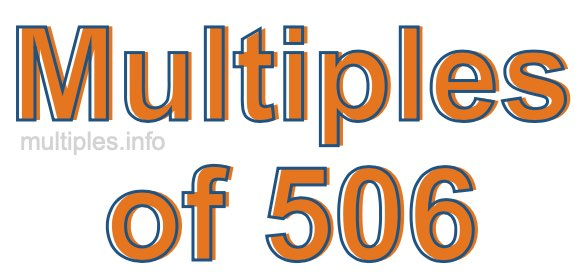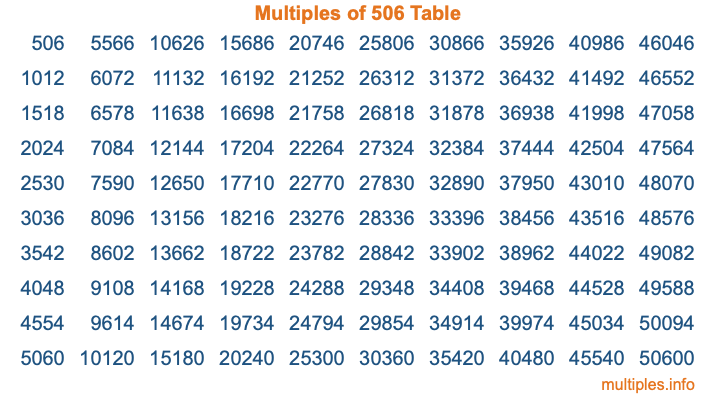Multiples of 506Welcome to the Multiples of 506 page. Here we will first teach you everything you will ever need to know about the multiples of 506, and then give you a study guide summary of everything we taught you to make sure you remember it all. Use this page to look up facts and learn information about the multiples of 506. This page will make you a multiples of five hundred six expert!

Definition of Multiples of 506
Multiples of 506 are all the numbers that when divided by 506 equal an integer. Each of the multiples of 506 are called a multiple. A multiple of 506 is created by multiplying 506 by an integer.

Therefore, to create a list of multiples of 506, you start with 1 multiplied by 506, then 2 multiplied by 506, then 3 multiplied by 506, and so on for as long as you want. Thus, the list of the first five multiples of 506 is 506, 1012, 1518, 2024, and 2530. To see a larger list of multiples of 506, see the printable image of Multiples of 506 further down on this page. We also have a category where you can choose any nth multiple of 506.

Multiples of 506 Checker
The Multiples of 506 Checker below checks to see if any number of your choice is a multiple of 506. In other words, it checks to see if there is any number (integer) that when multiplied by 506 will equal your number. To do that, we divide your number by 506. If the the quotient is an integer, then your number is a multiple of 506.

Is  a multiple of 506?

Least Common Multiple of 506 and ...
A Least Common Multiple (LCM) is the lowest multiple that two or more numbers have in common. This is also called the smallest common multiple or lowest common multiple and is useful to know when you are adding our subtracting fractions. Enter one or more numbers below (506 is already entered) to find the LCM.

Check out our LCM Calculator if you need more details about the Least Common Multiple or if you need the LCM for different numbers for adding and subtraction fractions.

nth Multiple of 506
As we stated above, 506 is the first multiple of 506, 1012 is the second multiple of 506, 1518 is the third multiple of 506, and so on. Enter a number below to find the nth multiple of 506.

th multiple of 506

Multiples of 506 vs Factors of 506
506 is a multiple of 506 and a factor of 506, but that is where the similarities end. All postive multiples of 506 are 506 or greater than 506. All positive factors of 506 are 506 or less than 506.

Below is the beginning list of multiples of 506 and the factors of 506 so you can compare:

Multiples of 506: 506, 1012, 1518, 2024, 2530, etc.

Factors of 506: 1, 2, 11, 22, 23, 46, 253, 506

As you can see, the multiples of 506 are all the numbers that you can divide by 506 to get a whole number. The factors of 506, on the other hand, are all the whole numbers that you can multiply by another whole number to get 506.

It's also interesting to note that if a number (x) is a factor of 506, then 506 will also be a multiple of that number (x).

Multiples of 506 vs Divisors of 506
The divisors of 506 are all the integers that 506 can be divided by evenly. Below is a list of the divisors of 506.

Divisors of 506: 1, 2, 11, 22, 23, 46, 253, 506

The interesting thing to note here is that if you take any multiple of 506 and divide it by a divisor of 506, you will see that the quotient is an integer.

Multiples of 506 Table
Below is an image of the first 100 multiples of 506 in a table. The table is in chronological order, column by column. The first column has the first ten multiples of 506, the second column has the next ten multiples of 506, and so on.The Multiples of 506 Table is also referred to as the 506 Times Table or Times Table of 506. You are welcome to print out our table for your studies.

Negative Multiples of 506
Although not often discussed or needed in math, it is worth mentioning that you can make a list of negative multiples of 506 by multiplying 506 by -1, then by -2, then by -3, and so on, to get the following list of negative multiples of 506:

-506, -1012, -1518, -2024, -2530, etc.

Multiples of 506 Summary
Below is a summary of important Multiples of 506 facts that we have discussed on this page. To retain the knowledge on this page, we recommend that you read through the summary and explain to yourself or a study partner why they hold true.

There are an infinite number of multiples of 506.

A multiple of 506 divided by 506 will equal a whole number.

506 divided by a factor of 506 equals a divisor of 506.

The nth multiple of 506 is n times 506.

The largest factor of 506 is equal to the first positive multiple of 506.

506 is a multiple of every factor of 506.

506 is a multiple of 506.

A multiple of 506 divided by a divisor of 506 equals an integer.

506 divided by a divisor of 506 equals a factor of 506.

Any integer times 506 will equal a multiple of 506.

Multiples of a Number
Here you can get the multiples of another number, all with the same attention to detail as we did for multiples of 506 on this page.

Multiples of
Multiples of 507
Did you find our page about multiples of five hundred six educational? Do you want more knowledge? Check out the multiples of the next number on our list!